# Auto-encoder & Classifier TensorFlow Models for Digit Classification

## Part 2: TensorFlow neural network implementation and training for classifying MNIST handwritten images.

This post will be covering the two models that were set up in TensorFlow to process MNIST digit data, how training was conducted, and finally how the results were converted into a tangible model to be leveraged downstream. This post is part of the TensorFlow + Docker MNIST Classifier series.

The Data Set (MNIST): This is one of the most popular machine learning data sets on the internet at the moment. It consists of tens of thousands of 28 x 28 labeled handwritten digits like the one below.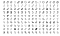some sample images from the MNIST data set

One of the key success criteria for this project was the use of multiple models in the final solution. The first model will be an auto-encoder to standardize the image data and the second model will classify it.

Features and targets

The features or digits will be passed through the model as 784-dimensional vectors with each element of the vector representing pixel intensity (white to black) of each pixel in the 28 x 28 image. Scaling was used on the feature data to improve performance converting the value range from [0.0, 255.0] to [0.0, 1.0] by dividing each value by 255.

Data set labels (targets) are a single dimensional vector with values ranging from 0–9, representing the 10 potential digit classes. In order to improve model performance and simplicity these were transformed into a 10 dimensional one-hot representation with each dimension representing the probability of the associated digit e.g.  -> [0, 0, 0, 0, 0, 1, 0, 0, 0, 0].

The model

One of the goals of this project was to implement a system with 2 models and I chose to use an auto-encoder as my first model and a basic classifier for my second as illustrate below.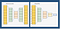2 model structure

Keras is used to simplify development and training, config files are used to store hyperparameters and file paths, and I developed a basic helper for loading MNIST image data as I am not using Keras for data loading.

SUPPORTING PYTHON OBJECTS

1. Config — Configuration file for the training
2. MNISTProcessor — MNIST data loader
3. DataWrapper — Object to handle training and testing data
4. Visualizer — Stored functions to help visualize results

The Auto-encoder: There is a lot of great material on the auto-encoder network online including the wiki entry here. In a nutshell, an auto-encoder is an unsupervised symmetrical neural network that compresses the feature vector into significantly fewer dimensions. The network is trained by using features as both the input and output of the network, teaching the filters to compress the features. One of the key uses of the auto-encoder is noise reduction and this is what it will be used for here.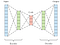Typical auto-encoder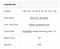Tanh — MSE — Adadelta

Using Keras we can implement the neural network using the following code.

The following function was created to help visualize the auto-encoder result.

After training my error loss was around 0.025 and you can see below what a few sample images looked like after being passed through the trained auto-encoder. The result could be improved but this should be satisfactory for our needs.

The Classifier: The second model will take the 784-dimensional vector output by the auto-encoder and classifying the data into one of the 10 possible digit values [0, 9]. A simple tanh activated deep neural network will be used.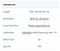Tanh — MSE — Adadelta

Keras was used to implement the classifier as well. We first load and process the image data through the auto-encoder before using it as the feature input for the training of the classifier.

Lessons learned

Poor initial model convergence — I wrote my initial code using the stand-alone Keras library, however, due to challenges of saving the models in a servable format I had to switch the tf.keras library instead. After my switch, my models flat out refused to converge during training. After many hours of debugging, I discovered that the `keras.optimizers.Adadelta` optimizer uses a default starting learning rate of 1.0, whereas the tf.keras.optimizersAdadelta optimizer initializes with a learning rate of 0.001. Forcing the learning rate addressed this issue for me and you can see this reflected in my code.

For the lazy

My results can be reproduced with the following commands:

ou should now see the production models under `models/autoencoder/production/1` and `models/classifier/production/1` that looks like this:

The entire TensorFlow GitHub repository along with complete instructions on running the model can be found here. Now that we have both the auto-encoder and classifier models generated we can take a look at deploying them via TensorFlow serving, which I will do in my next post.

Here is a summary of the components involved in this project:

1. Introduction
2. The Models : git repo → tf-mnist-project
3. Serving Models : git repo → tf-serving-mnist-project
4. The User Interface : git repo → angular-mnist-project

Serial tinkerer and digital architect

## More from Andrew Didinchuk

Serial tinkerer and digital architect